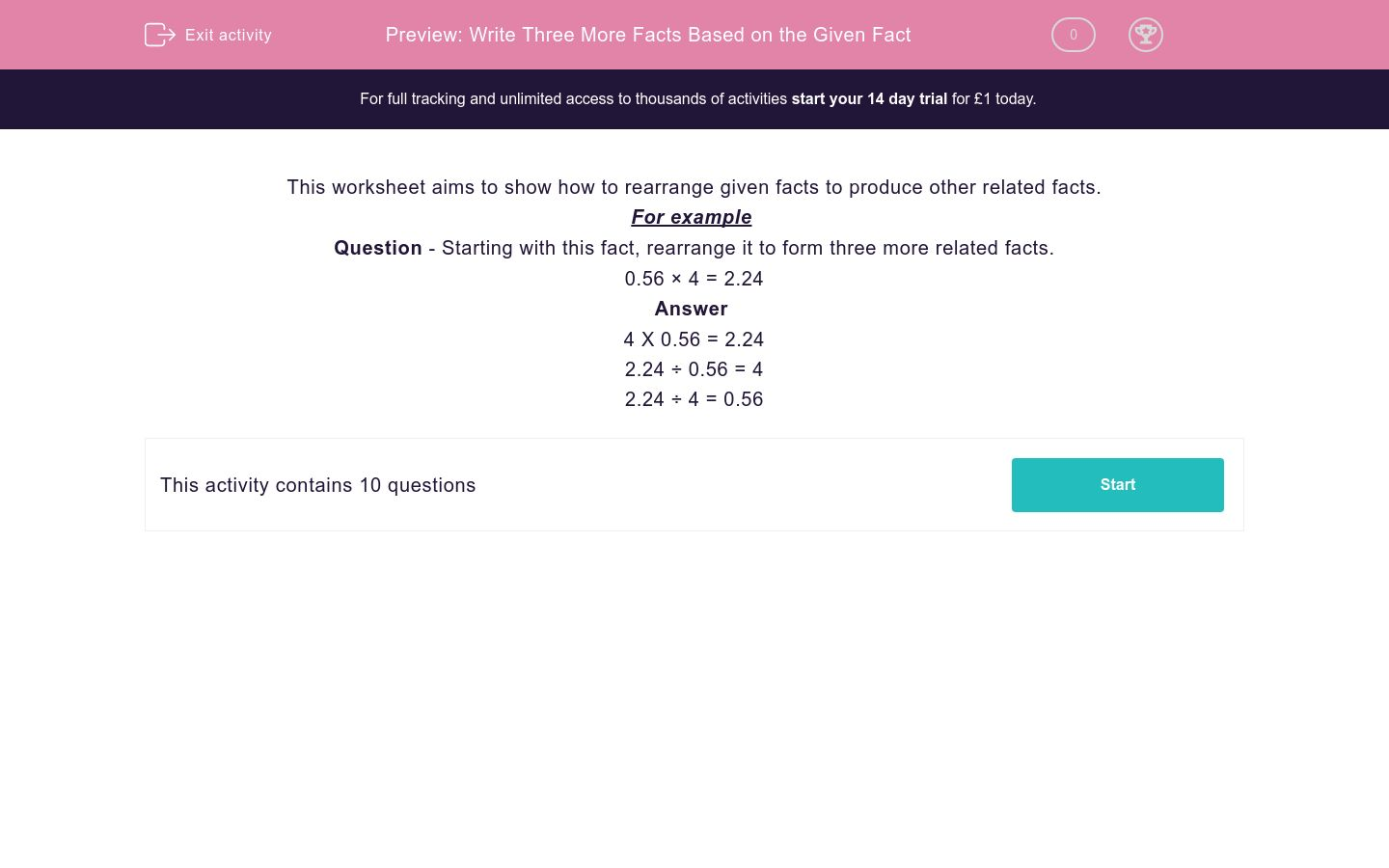# Write Three More Facts Based on the Given Fact

In this worksheet, students understand how multiplication and division facts are connected.Key stage:  KS 2

Curriculum topic:   Maths and Numerical Reasoning

Curriculum subtopic:   Mixed Problems

Difficulty level:### QUESTION 1 of 10

This worksheet aims to show how to rearrange given facts to produce other related facts.

For example

Question - Starting with this fact, rearrange it to form three more related facts.

0.56 × 4 = 2.24

4 X 0.56 = 2.24

2.24 ÷ 0.56 = 4

2.24 ÷ 4 = 0.56

Which three facts can be produced from this one?

72 ÷ 9 = 8

72 x 9 = 8

72 ÷ 8 = 9

8 x 9 = 72

8 ÷ 72 = 9

9 x 8 = 72

Which three facts can be produced from this one?

1.92 ÷ 3 = 0.64

1.92 ÷ 0.64 = 3

0.64 x 3 = 1.92

0.64 ÷ 3 = 1.92

3 x 0.64 = 1.92

1.92 x 0.64 = 3

Which three facts can be produced from this one?

5.88 ÷ 7 = 0.84

0.84 x 5.88 = 7

0.84 x 7 = 5.88

5.88 ÷ 0.84 = 7

7 x 0.84 = 5.88

7 ÷ 5.88 = 0.84

Which three facts can be produced from this one?

0.99 ÷ 6 = 0.165

0.99 x 0.165 = 6

0.99 x 6 = 0.165

0.99 ÷ 0.165 = 6

6 x 0.165 = 0.99

0.165 x 6 = 0.99

Which three facts can be produced from this one?

0.14 X 6 = 0.84

6 x 0.14 = 0.84

6 x 0.84 = 0.14

6 ÷ 0.84 = 0.14

0.84 ÷ 0.14 = 6

0.84 ÷ 6 = 0.14

Which three facts can be produced from this one?

24 x 18 = 432

432 ÷ 18 = 24

432 x 18 = 24

24 ÷ 18 = 432

432 ÷ 24 = 18

18 x 24 = 432

Which three facts can be produced from this one?

0.38 × 1 = 0.38

1 ÷ 0.38 = 0.38

0.38 x 0.38 = 1

0.38 ÷ 0.38 = 1

0.38 ÷ 1 = 0.38

1 x 0.38 = 0.38

Which three facts can be produced from this one?

0.16 × 5 = 0.8

0.16 ÷ 0.8 = 5

0.8 ÷ 0.16 = 5

0.8 ÷ 5 = 0.16

0.16 x 0.8 = 5

5 x 0.16 = 8

Which three facts can be produced from this one?

0.55 × 12 = 6.6

12 x 0.55 = 6.6

6.6 ÷ 12 = 0.55

6.6 ÷ 0.55 = 12

6.6 x 0.55 = 12

12 ÷ 6.6 = 0.55

Write three facts can be produced from this one?

10.58 × 124 = 1311.92

1311.92 ÷ 124 = 10.58

124 ÷ 10.58 = 1311.92

1311.92 ÷ 10.58 = 124

1311.92 x 10.58 = 124

124 x 10.58 = 1311.92

• Question 1

Which three facts can be produced from this one?

72 ÷ 9 = 8

72 ÷ 8 = 9
8 x 9 = 72
9 x 8 = 72
• Question 2

Which three facts can be produced from this one?

1.92 ÷ 3 = 0.64

1.92 ÷ 0.64 = 3
0.64 x 3 = 1.92
3 x 0.64 = 1.92
• Question 3

Which three facts can be produced from this one?

5.88 ÷ 7 = 0.84

0.84 x 7 = 5.88
5.88 ÷ 0.84 = 7
7 x 0.84 = 5.88
• Question 4

Which three facts can be produced from this one?

0.99 ÷ 6 = 0.165

0.99 ÷ 0.165 = 6
6 x 0.165 = 0.99
0.165 x 6 = 0.99
• Question 5

Which three facts can be produced from this one?

0.14 X 6 = 0.84

6 x 0.14 = 0.84
0.84 ÷ 0.14 = 6
0.84 ÷ 6 = 0.14
• Question 6

Which three facts can be produced from this one?

24 x 18 = 432

432 ÷ 18 = 24
432 ÷ 24 = 18
18 x 24 = 432
• Question 7

Which three facts can be produced from this one?

0.38 × 1 = 0.38

0.38 ÷ 0.38 = 1
0.38 ÷ 1 = 0.38
1 x 0.38 = 0.38
• Question 8

Which three facts can be produced from this one?

0.16 × 5 = 0.8

0.8 ÷ 0.16 = 5
0.8 ÷ 5 = 0.16
5 x 0.16 = 8
• Question 9

Which three facts can be produced from this one?

0.55 × 12 = 6.6

12 x 0.55 = 6.6
6.6 ÷ 12 = 0.55
6.6 ÷ 0.55 = 12
• Question 10

Write three facts can be produced from this one?

10.58 × 124 = 1311.92

1311.92 ÷ 124 = 10.58
1311.92 ÷ 10.58 = 124
124 x 10.58 = 1311.92
---- OR ----

Sign up for a £1 trial so you can track and measure your child's progress on this activity.

### What is EdPlace?

We're your National Curriculum aligned online education content provider helping each child succeed in English, maths and science from year 1 to GCSE. With an EdPlace account you’ll be able to track and measure progress, helping each child achieve their best. We build confidence and attainment by personalising each child’s learning at a level that suits them.

Get started